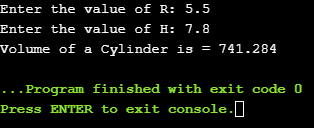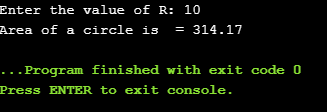# Constants In C++: Literal Constant, Symbolic Constant, Const Qualifier, Define Directive

(Last Updated On: November 18, 2020)

## Constants:

Constants in C++ – The quantities that cannot change their value during the execution of a program are called constants. We would never want to change the value of Pi, Speed of light, etc.

Constants in C++ can be divided into two basic types:

• Literal Constant in C++
• Symbolic Constant in C++

We will talk in detail about these two types and I will try to explain the Literal Constant and Symbolic Constant with help of some example codes. So, first let’s start with the Literal Constant in C++.

## Literal Constant in C++:

The word ‘literal ‘ means accurate or exact. A literal is a constant in C++ having independent value that is used in a program source code. For example, the value 10, 10.3 and “Excellent” represent literal constants in C++.

Literal constants in C++ are further divided into:

• Integer constants in C++
• Floating-point constants in C++
• Character constants in C++
• String constants in C++

### Integer Constants in C++:

the numeric value that have no decimal part are known as integer constants. The numeric value 10, -16, 340, 89 are example of integer constants in C++. While using the integer constants in C++ , these integer constants must lie within the range of integer. The + and – signs can also used with integer constants. Usually, the integer constants are used in arithmetic expression.

### Floating-point Constants in C++:

The numeric values that have decimal part are known as float-point constants. They may be positive or negative. The numeric values 5.143, 6.0 and -7.1 are some examples of floating point constants in C++.

The number 8.214F is also an example of floating point constants. The letter F in the number indicates that its data type is float. If the letter “F” is deleted then the data type of number will be considered as “double”. We can also specify or declare the data type as “long double” by simply using the letter L. for example, the number 56.23L indicates that this number is the long double floating point constants in C++.

We can also write floating point constants using exponential notation. Usually large values are written in exponential notation. For example, 70000000.0 can be written as 70.0E6 in exponential notation. Similarly, 16.72 x 1019 can be written as 16.72E19. the letter E represents the exponent.

### Character Constants in C++:

You may already be familiar with the Character constants in C++ which are always enclosed in single quotation marks. A single character which may be an alphabet, a digit or even a special character. For example, ‘x’, ‘y’, ‘+’, ‘a’ and ‘9’ represent character constants in C++.

### String Constants in C++:

A sequence of characters enclosed in double quotation marks is known as string constant or string literal. A string constant is C++ programming language is considered as the single token by the C++ compiler. For example, “Intruder dected”, “04657654”, MSC-454” represent string constants in C++. If you check my articles on the Arduino and GSM, you will find I have been using the string constants for sending text messages to specific cell phone numbers.

## Symbolic Constants in C++:

The symbolic constants are constant identifier. Before the symbolic constants are used they be initialized. So, after a symbolic constant is initialized as per the rules, its value cannot be changed during program execution. Every programming language has its own style of declaring the constants, in C++ programming language the symbolic constants can be declared in two ways:

• Using ‘const’ keyword
• Using ‘define’ directive

### The ‘const’ Keyword in C++:

The ‘const’ keyword is used to declare constant identifier. The constant identifier is declared in the same exact way as variable is declared except that a keyword “const” is used to specify a constant identifier. A value must be assigned to constant identifier at the time of its declaration. Once a value is assigned, it cannot be changed during program execution. For example, to declare a variable PI and to assign value 3.1417 by using ‘const’ keyword, the statement is written as:

const float PI=3.1417;

PI is the variable name, its type is float and this is a constant. So, currently a value of 3.1417 is stored in the variable PI. This value will remain the same throughout the program. Let me explain this with the help of an example.

#### Example: write a program that computes the volume of a cylinder using const qualifier. The formula to find the volume to cylinder is: volume. The value of  is 3.1417:### The ‘define’ Directive:

The ‘define’ is a preprocessor directive. It is used to define a constant identifier known as constant macro. A constant macro is an identifier, which is assigned a particular constant in C++ value. like other preprocessor directives, the define directive is also used at the beginning of the program.

The general syntax of ‘define’ directive is as follows:

#define identifier expression;

Where

Identifier:

It indicates the name of constant identifier to which the constant value is to be assigned. This name cannot be used again in the program as variable name or function name etc.

Expression:

It indicates the constant value that is to be assigned to the identifier. It may be a constant value, a string or an string or an arithmetic expression.

In the following statement, PI is assigned value 3.141592 and ‘city’ is assigned value ‘uk’.

#define PI 3.141593

#define city uk

## Difference Between define and const in C++:

The difference between’define’ directive and ‘const’ qualifier is as follows:

 Define Directive const Qualifier It is used as preprocessor directive. It is used as statement. It is not terminated with semicolon (;) It is terminated with semicolon. Data type of constant identifier is not specified Data type of constant identifier is specified.

### Example: write program that computes the area of a circle using constant define directive.

To compute the area of a circle you should know what formula is used. The formula to find the area of a circle is: area. The value of  is 3.1417.